# Conversions Guide For Square And Round Cake Tins

Whenever the terms square and round cake tins enter a conversation, you can be assured that a question related to either of the following with crop up:

Question 1. Volumes
For a given size of tin, which one will hold a larger volume?

Question 2. Recipes
How do I adjust a recipe from a square to round cake tin or visa versa?

### Easy Conversions Guide For Square and Round Cake Tins

An easily remembered conversions guide for square and round cake tins would be the following:

A round cake tin will hold approximately 25% or a 1/4 less than a square tin

and

Use a round cake tin that is one size larger than a square tin for a given recipe

Therefore, we can make a simple quick comparison chart (in inches) for adjusting a recipe from a square to round cake tin or visa versa.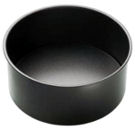5″ 4″ 6″ 5″ 7″ 6″ 8″ 7″ 9″ 8″ 10″ 9″ 11″ 10″ 12″ 11″ 13″ 12″ 14″ 13″ 15″ 14″ 16″ 15″

For practical usage that covers what you need to know.

……however, for anyone interested in the mathematics, the following might be of interest!

### Advanced Conversions Guide For Square and Round Cake Tins

 A1.Volumes Please note: For comparison, we will use a depth of 4 inches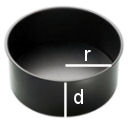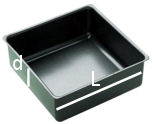Formula for Volume 3.14r2d L2d Explanation 3.14 is an approximation for pi r= radius is half of the diameter r2= r x r d= depth (4 inches in the example) Because it is a square, the length (L) is the same as the width L2= L x L d= depth (4 inches in the example) Example: 6 inch tin If we use the formula above then 6 inch tin has a radius (r) of 3 3.14r2d 3.14 x 32 x 4= 3.14 x 9 x 4= 113.04 Using the formula above then 6 inch tin has a length (L) of 6 L2d 62 x 4 = 36 x 4 = 144

Therefore, comparing the round cake tin to the square cake tin, we have:

113.04 compared to 144 which can be shown as
113.04 : 144 or
1: 144 ÷ 113.04
which equals approximately 1: 1.27

Thus, the square cake tin has a volume about 27% larger (which we simplified as approximately 25%) than the round cake tin for the ‘same’ size of tin and depth.

 A2.Recipes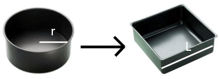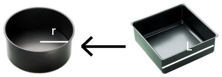Explanation 6″ round cake tin means the tin’s diameter is 6 Therefore it has a radius (r) of 3 Area of circle= 3.14r2 3.14 (32) = 3.14 (3 x 3)= 3.14 x 9= 28.26 square inches We now to need find the length (L) for the square cake tin i.e. the ‘size’ of the square cake tin Area of square= L2 and we know the area to be 28.26 from above Therefore, L2= 28.26 We can find L by obtaining the square root of 28.26 L is therefore approximately 5.32 So, if we round 5.32 to the nearest inch, we arrive at 5 5″ square cake tin means the length (L) is 5 Area of square = L2 5 x 5 = 25 square inches We now need to find the diameter of the round cake tin i.e. the ‘size’ of the round cake tin Area of circle = 3.14 r2 and we know the area to be 25 from above Therefore, if we re-arrange the above formula we get r2 = Area of circle ÷  3.14 r2 = 25 ÷ 3.14 r2= 7.96 We can find r by obtaining the square root of 7.96 r is therefore approximately 2.82 Finally, the diameter of the round tin is 2 x r   = 2 x 2.82 = 5.64 So, if we round 5.64 to the nearest inch, we arrive at 6 Summary 6″ round cake tin is equivalent to a 5″ square cake tin 5″ square cake tin is equivalent to a 6″ round cake tin

Therefore, the key points you should take from this conversions guide for square and round cake tins are:

A round cake tin will hold approximately 25% or a 1/4 less than a square tin

and

Use a round cake tin that is one size larger than a square tin for a given recipe

Other articles of interest:
Care of Cake Tins

This website is a participant in the Amazon Services LLC Associate Program, an affiliate advertising program designed to provide a means for sites to earn advertising fees by advertising and linking products to various Amazon properties.